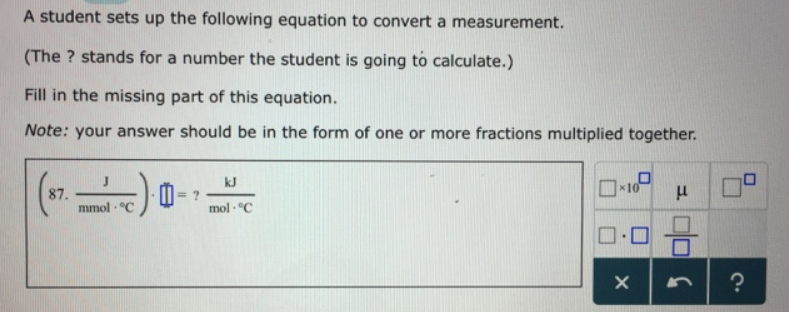# Problem: A student sets up the following equation to convert a measurement. (The ? stands for a number the student is going to calculate.) Fill in the missing part of this equation. Note: your answer should be in the form of one or more fractions multiplied together.

###### FREE Expert Solution
88% (478 ratings)###### Problem Details

A student sets up the following equation to convert a measurement.

(The ? stands for a number the student is going to calculate.)

Fill in the missing part of this equation.

Note: your answer should be in the form of one or more fractions multiplied together.